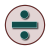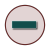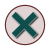Games
Problems
Go Pro!

# Division

Lesson Plans > Mathematics > Division

# Featured Lesson PlansLesson plan for elementary mathematics teacher teaching students about division with one and zeroDivision Unit: Writing A Number Sentence - solve problems and determine whether multiplication or division should be usedLesson plan for mathematics teacher teaching division. Focuses on division by two and division by 5Lesson plan for third grade mathematics teacher, teaching division by three and four.Learning to Use Division; A Pre-Service Teacher's Look - student submitted unit from Elementary Mathematics Methods CourseDivision - Meaning, Manipulatives and Relationships. Third Grade lesson plan unit for understanding divisionRelating Division And Subtraction Lesson Plan - connecting subtraction and division.Connecting Division And Multiplication Lesson Plan - Understanding inverse relationships and fact families

# Full Directory Listing

Connecting Division And Multiplication, Dividing By 2 and 5 (Two And Five), Dividing By 3 and 4 (Three and Four) Lesson Plan, Dividing With 1 and 0 (One and Zero) Lesson Plan, Division - Meaning, Manipulatives and Relationships, Division Unit: Writing A Number Sentence, Learning to Use Division (Division Unit Rationale), Relating Division And Subtraction Lesson PlanLike us on Facebook to get updates about new resources